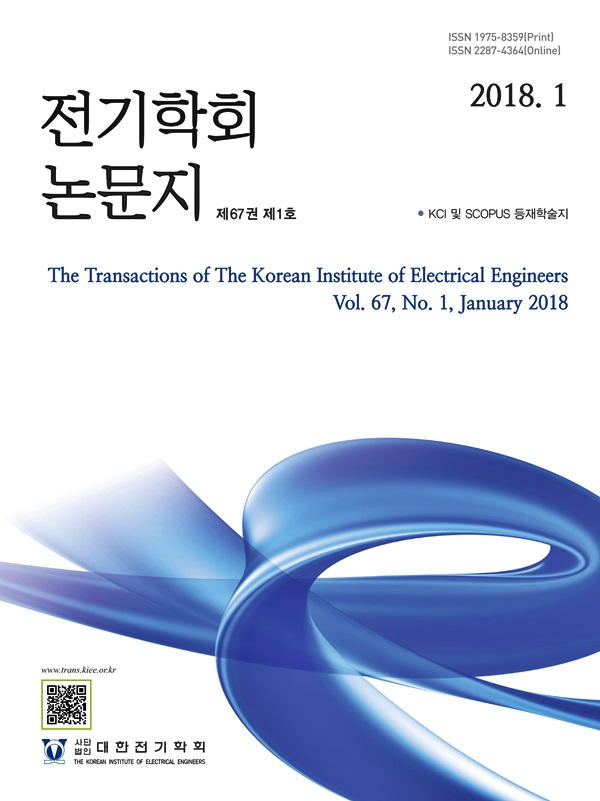Mobile QR CodeThe Transactions of the Korean Institute of Electrical Engineers

ISO Journal TitleTrans. Korean. Inst. Elect. Eng.
Title Coupling Method of Circuit Equation and Magnetic Field Distribution for Characterization of Induction Motors by Coordinate Transformation 김영선(Young-Sun Kim) https://doi.org/10.5370/KIEE.2021.70.12.2058 pp.2058-2063 1975-8359 Circuit Equation; Coordinate Transformation; Finite element analysis; Induction motor; Lorentz force The induction motors are continuously used because of their efficiency and convenience in maintenance. In this paper, a time step analysis method that can be effectively applied to the analysis of three-phase and single-phase induction motors has been proposed.The unknown variables in the differential equations of the circuit are the currents flowing through the rotor bars when analyzing an electric motor. The variables are coupled with the distributed magnetic flux density in the air gap instead of the magnetic inductance matrix while applying Kirchhoff’s law and Faraday’s induction law. Two calculated patterns for magnetic flux densities are necessary in the analysis. The first pattern is given by an ideal stator’s winding distribution, whereas the second one is produced by currents flowing through the rotor conductor bar with unit value and is calculated using the conventional finite element method(FEM). The formulated equation set is applied for a simple three-phase and single-phase example model and the resultant torque-speed curve is presented in this paper.